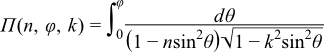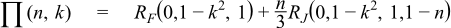#Boost C++ Libraries

...one of the most highly regarded and expertly designed C++ library projects in the world.

This is the documentation for an old version of boost. Click here for the latest Boost documentation.

#### Elliptic Integrals of the Third Kind - Legendre Form

###### Synopsis

```#include <boost/math/special_functions/ellint_3.hpp>
```

```namespace boost { namespace math {

template <class T1, class T2, class T3>
calculated-result-type ellint_3(T1 k, T2 n, T3 phi);

template <class T1, class T2, class T3, class Policy>
calculated-result-type ellint_3(T1 k, T2 n, T3 phi, const Policy&);

template <class T1, class T2>
calculated-result-type ellint_3(T1 k, T2 n);

template <class T1, class T2, class Policy>
calculated-result-type ellint_3(T1 k, T2 n, const Policy&);

}} // namespaces
```
###### Description

These two functions evaluate the incomplete elliptic integral of the third kind Π(n, φ, k) and its complete counterpart Π(n, k) = E(n, π/2, k).The return type of these functions is computed using the result type calculation rules when the arguments are of different types: when they are the same type then the result is the same type as the arguments.

```template <class T1, class T2, class T3>
calculated-result-type ellint_3(T1 k, T2 n, T3 phi);

template <class T1, class T2, class T3, class Policy>
calculated-result-type ellint_3(T1 k, T2 n, T3 phi, const Policy&);
```

Returns the incomplete elliptic integral of the third kind Π(n, φ, k):Requires -1 <= k <= 1 and n < 1/sin2(φ), otherwise returns the result of domain_error (outside this range the result would be complex).

The final Policy argument is optional and can be used to control the behaviour of the function: how it handles errors, what level of precision to use etc. Refer to the policy documentation for more details.

```template <class T1, class T2>
calculated-result-type ellint_3(T1 k, T2 n);

template <class T1, class T2, class Policy>
calculated-result-type ellint_3(T1 k, T2 n, const Policy&);
```

Returns the complete elliptic integral of the first kind Π(n, k):Requires -1 <= k <= 1 and n < 1, otherwise returns the result of domain_error (outside this range the result would be complex).

The final Policy argument is optional and can be used to control the behaviour of the function: how it handles errors, what level of precision to use etc. Refer to the policy documentation for more details.

###### Accuracy

These functions are computed using only basic arithmetic operations, so there isn't much variation in accuracy over differing platforms. Note that only results for the widest floating point type on the system are given as narrower types have effectively zero error. All values are relative errors in units of epsilon.

Table 46. Errors Rates in the Elliptic Integrals of the Third Kind

Significand Size

Platform and Compiler

Π(n, φ, k)

Π(n, k)

53

Win32 / Visual C++ 8.0

Peak=29 Mean=2.2

Peak=3 Mean=0.8

64

Red Hat Linux / G++ 3.4

Peak=14 Mean=1.3

Peak=2.3 Mean=0.8

113

HP-UX / HP aCC 6

Peak=10 Mean=1.4

Peak=4.2 Mean=1.1

###### Testing

The tests use a mixture of spot test values calculated using the online calculator at functions.wolfram.com, and random test data generated using NTL::RR at 1000-bit precision and this implementation.

###### Implementation

The implementation for Π(n, φ, k) first siphons off the special cases:

Π(0, φ, k) = F(φ, k)

Π(n, π/2, k) = Π(n, k)

andThen if n < 0 the relations (A&S 17.7.15/16):are used to shift n to the range [0, 1].

Then the relations:

Π(n, -φ, k) = -Π(n, φ, k)

Π(n, φ+mπ, k) = Π(n, φ, k) + 2mΠ(n, k) ; n <= 1

Π(n, φ+mπ, k) = Π(n, φ, k) ; n > 1 

are used to move φ   to the range [0, π/2].

The functions are then implemented in terms of Carlson's integrals using the relations:andI haven't been able to find a literature reference for this relation, but it appears to be the convention used by Mathematica. Intuitively the first 2 * m * Π(n, k) terms cancel out as the derivative alternates between +∞ and -∞.